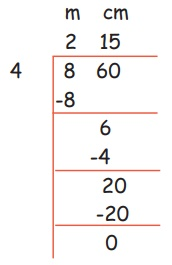Home | | Maths 5th Std | Division of length

# Division of length

Measurements : Division of length

Division

Example 1

(i) 84 m 40 cm ÷ 484 m 40 cm ÷ 4 = 21 m 10 cm

(ii) 360 km 540 m ÷ 9Answer = 40 km 060 m

Try this

a.750 m 45cm ÷ 5 = 150 m 09 cm

b. 49 km 630m ÷ 7 = 7 km 090 m

c. 770 km 550 m ÷11 = 70 km 050 m

Example 2

If the total length of 4 pieces of clothes is 8 m 60 cm. What is the length of one piece of cloth?

Solution:

Total length of cloth = 8m 60 cm

Length of one piece = 8 m 60 cm ÷ 4Length of one piece = 2 m 15 cm

Length of one piece = 2 m 15cm

Tags : Measurements | Term 1 Chapter 4 | 5th Maths , 5th Maths : Term 1 Unit 4 : Measurements
Study Material, Lecturing Notes, Assignment, Reference, Wiki description explanation, brief detail
5th Maths : Term 1 Unit 4 : Measurements : Division of length | Measurements | Term 1 Chapter 4 | 5th Maths